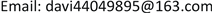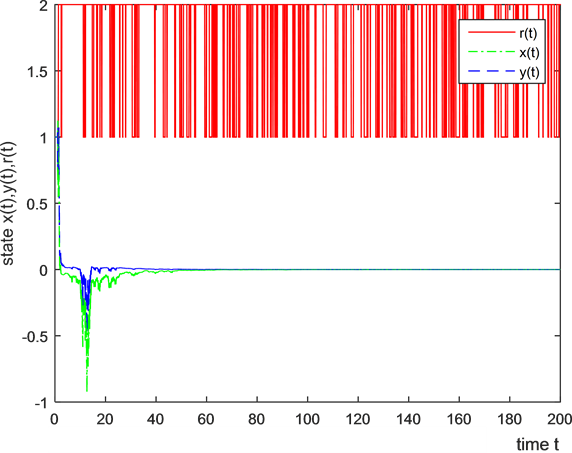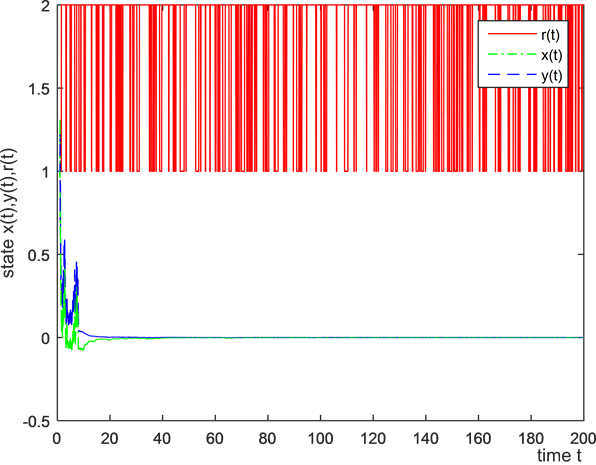﻿ 马尔可夫调制的Kaldor-Kalecki模型的随机稳定性仿真研究 Simulation of Stochastic Stability of Kaldor-Kalecki Model with Markovian Switching

World Economic Research
Vol.06 No.04(2017), Article ID:23119,6 pages
10.12677/WER.2017.64013

Simulation of Stochastic Stability of Kaldor-Kalecki Model with Markovian Switching

Lujun Zhou

College of Economics, Hunan Agricultural University, Changsha HunanReceived: Nov. 28th, 2017; accepted: Dec. 15th, 2017; published: Dec. 22nd, 2017ABSTRACT

This paper studies that the economic system is in the random environment which has Markov chain with two discrete values. By using the method of stochastic stability and the given parameters, the stability of the solution of the random Kaldor-Kalecki model with Markovian Switching is discussed, and the illustrative simulation is obtained.

Keywords:Kaldor-Kalecki Model, Stochastic Differential Equation, Delayed Time, Markovian Switching, Stability1. 引言

2. 预备知识及模型构建

Mao (2001)研究了如下的马尔可夫调制的随机微分方程  :

$\text{d}x\left(t\right)=f\left(x\left(t\right),x\left(t-\tau \right),t,r\left(t\right)\right)\text{d}t+g\left(x\left(t\right),x\left(t-\tau \right),t,r\left(t\right)\right)\text{d}w\left(t\right)$

$|\cdot |$ 表示Rn中欧氏范数，假如A是一个矩阵(用AT表示A的转置)，则定义其迹范数为 $|A|=\sqrt{trace\left({A}^{\text{T}}A\right)}$ ，定义算子范数为 $‖A‖=\mathrm{sup}\left\{|Ax|:|x|=1\right\}$ 。设 $r\left(t\right),t\ge 0$ 是定义在完备概率空间

$\left(\Omega ,F,{\left\{{F}_{t}\right\}}_{t\ge 0},P\right)$ 上的右连续马尔可夫链，取两个值的状态空间 $S=\left\{1,2,\cdots ,N\right\}$ ，且具有生成元 $\Gamma ={\left({\gamma }_{ij}\right)}_{N×N}$ ，其中

$P\left\{r\left(t+\Delta \right)=j|r\left(t\right)=i\right\}=\left\{\begin{array}{l}{\gamma }_{ij}\Delta +o\left(\Delta \right),\text{\hspace{0.17em}}\text{\hspace{0.17em}}\text{\hspace{0.17em}}\text{\hspace{0.17em}}\text{\hspace{0.17em}}\text{\hspace{0.17em}}\text{ }\text{\hspace{0.17em}}i\ne j\\ 1+{\gamma }_{ij}\Delta +o\left(\Delta \right),\text{\hspace{0.17em}}\text{\hspace{0.17em}}\text{\hspace{0.17em}}i=j\end{array}$

$V\in {C}^{2,1}\left({R}^{n}×{R}_{+}×S;{R}_{+}\right)$ ，定义算子 $LV$ 如下

$\begin{array}{c}LV\left(x,t,i\right)={V}_{t}\left(x,t,i\right)+{V}_{x}\left(x,t,i\right)f\left(x,y,t,i\right)\\ \text{\hspace{0.17em}}\text{\hspace{0.17em}}+\frac{1}{2}trace\left[{g}^{\text{T}}\left(x,y,t,i\right){V}_{xx}\left(x,t,i\right)g\left(x,y,t,i\right)\right]+\sum _{j=1}^{N}{\gamma }_{ij}V\left(x,t,j\right),\end{array}$

Kaldor (1940)分析了Kaldor模型  ，

$\left\{\begin{array}{l}\stackrel{˙}{Y}\left(t\right)=\alpha \left(I\left(Y,K\right)-S\left(Y,K\right)\right),\\ \stackrel{˙}{K}\left(t\right)=I-\delta K.\end{array}$ (1)

Kalecki (1935)通过将投资依赖于投资决定做出的时机的收入和投资完成的时机的资本存量，考虑到了资本积累方程 

$\stackrel{˙}{K}\left(t\right)=I\left(Y\left(t-\tau \right),K\right)-\delta K$

M. Szydlowski and Krawiee A. (2001, 2001)分析了Kaldor-Kalecki模型   ，

$\left\{\begin{array}{l}\stackrel{˙}{Y}\left(t\right)=\alpha \left(I\left(Y,K\right)-S\left(Y,K\right)\right),\\ \stackrel{˙}{K}\left(t\right)=I\left(Y\left(t-\tau \right),K\right)-\delta K.\end{array}$ (2)

$I\left(Y,K\right)=\gamma I\left(Y\right)-\gamma K$$I\left(Y\right)=\upsilon Y$$\gamma >0$$I\left(Y\left(t-\tau \right),K\right)=\gamma I\left(Y\left(t-\tau \right)\right)-\gamma K=\gamma \upsilon Y\left(t-\tau \right)-\gamma K$

$\left\{\begin{array}{l}\stackrel{˙}{Y}\left(t\right)=\alpha \left(\gamma \upsilon -s\right)Y\left(t\right)-\alpha \gamma K\left(t\right),\\ \stackrel{˙}{K}\left(t\right)=\gamma \upsilon Y\left(t-\tau \right)-\left(\gamma +\delta \right)K\left(t\right).\end{array}$ (3)

$\text{d}X\left(t\right)=\left(AX\left(t\right)+BX\left(t-\tau \right)\right)\text{d}t$

$\left\{\begin{array}{l}\stackrel{˙}{Y}\left(t\right)=\left(\alpha \left(\gamma \upsilon -s\right)+{\sigma }_{1}w\left(t\right)\right)Y\left(t\right)+\left(-\alpha \gamma +{\sigma }_{2}w\left(t\right)\right)K\left(t\right),\\ \stackrel{˙}{K}\left(t\right)=\left(\gamma \nu +{\sigma }_{3}w\left(t\right)\right)Y\left(t-\tau \right)+\left(-\left(\gamma +\delta \right)+{\sigma }_{4}w\left(t\right)\right)K\left(t\right).\end{array}$ (4)

$⇒\left\{\begin{array}{l}\text{d}Y\left(t\right)=\left(\alpha \left(\gamma \upsilon -s\right)Y\left(t\right)+\left(-\gamma \right)K\left(t\right)\right)\text{d}t+\left({\sigma }_{1}Y\left(t\right)+{\sigma }_{2}K\left(t\right)\right)\text{d}w\left(t\right),\\ \text{d}K\left(t\right)=\left(\left(\gamma \upsilon \right)Y\left(t-\tau \right)-\left(\gamma +\delta \right)K\left(t\right)\right)\text{d}t+\left({\sigma }_{3}Y\left(t-\tau \right)+{\sigma }_{4}K\left(t\right)\right)\text{d}w\left(t\right).\end{array}$ (5)

$\text{d}X\left(t\right)=\left(AX\left(t\right)+BX\left(t-\tau \right)\right)\text{d}t+\left(CX\left(t\right)+DX\left(t-\tau \right)\right)\text{d}w\left(t\right)$ (6)

$\text{d}X\left(t\right)=\left(A\left(r\left(t\right)\right)X\left(t\right)+B\left(r\left(t\right)\right)X\left(t-\tau \right)\right)\text{d}t+\left(C\left(r\left(t\right)\right)X\left(t\right)+D\left(r\left(t\right)\right)X\left(t-\tau \right)\right)\text{d}w\left(t\right),$ (7)

3. 案例仿真

$\begin{array}{l}{\alpha }_{1}=0.011,{\gamma }_{1}=0.018,{\delta }_{1}=0.049,{s}_{1}=0.024,{\upsilon }_{1}={s}_{1}\ast \left(1/{\gamma }_{1}+1/{\delta }_{1}\right);\\ {\alpha }_{2}=0.012,{\gamma }_{2}=0.017,{\delta }_{2}=0.056,{s}_{2}=0.031,{\upsilon }_{2}={s}_{2}\ast \left(1/{\gamma }_{2}+1/{\delta }_{2}\right);\end{array}$

${C}_{1}=\left(\begin{array}{cc}0.556& 0.635\\ 0& 0.621\end{array}\right),{C}_{2}=\left(\begin{array}{cc}0.548& 0.624\\ 0& 0.671\end{array}\right),\text{\hspace{0.17em}}{D}_{1}=\left(\begin{array}{cc}0& 0\\ 0.246& 0\end{array}\right),{D}_{2}=\left(\begin{array}{cc}0& 0\\ 0.225& 0\end{array}\right)$

$\Gamma =\left(\begin{array}{cc}-3& 3\\ 1& -1\end{array}\right),\tau =0.2$Figure 1. Solution x(t), y(t) of sde and r(t) under the first sampleFigure 2. Solution x(t), y(t) of sde and r(t) under the second sampleFigure 3. Solution x(t), y(t) of sde and r(t) under the third sampleFigure 4. Solution x(t), y(t) of sde and r(t) under the fourth sample

Simulation of Stochastic Stability of Kaldor-Kalecki Model with Markovian Switching[J]. 世界经济探索, 2017, 06(04): 109-114. http://dx.doi.org/10.12677/WER.2017.64013

1. 1. Mao, X., Matasov, A. and Piunovskiy, A.B. (2000) Stochastic Differential Delay Equations with Markovian Switching. Bernoulli, 6, 73-90

2. 2. Kalecki, M. (1935) A Macrodynamic Theory of Business Cycles. Econometrica, 3, 327-344.

3. 3. Kaldor, N. (1940) A Model of the Trade Cycle. Economic Journal, 50, 78-92.

4. 4. Szydlowski, M.A. and Krawiee A. (2001) The Kaldor-Kalecki Model of Business Cycle as a Two-Dimensional Dynamical System. Journal of Nonlinear Mathematical Physics, 8, 266-271.

5. 5. Krawiee, A. and Szydlowski M.A. (1999) The Kaldor-Kalecki Business Cycle Model. Annals of Operations Research, 89, 89-100.